Lagrange Points and Methods for Observing Extrasolar Trojan Planets

The Three-Body Problem

The equations of motion for two gravitationally interacting bodies can be determined analytically with some effort. Unfortunately, the more complicated three-body problem cannot generally be solved in such a straightforward manner . Some interesting information can be gained, however, by exploring the three-body system in the limit where the mass of the third body is very small. This is often called the restricted three-body problem. With this simplification, the heavier two masses move about each other in the characteristic elliptical orbits of Keplerian motion unperturbed by the smaller third mass. If, in addition, we assume that the orbits of the heavier masses are circular, the problem becomes even more approachable.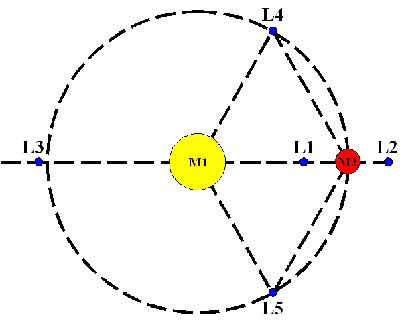Fig. 1: Approximate Locations of the five Lagrange Points, where M1 is much greater than M2. Adapted from .

Both of these simplifications apply to a wide range of astronomical systems of interest. For instance, if the three bodies are the Sun, the Earth, and an artificial satellite, then the satellite has a very small mass, while the Earth's orbit is nearly circular. Similarly, the three-body problem consisting of a star, a Jupiter-sized planet, and an Earth-sized planet is well-approximated by the restricted three-body problem.

Lagrange Points

Even with the simplifications discussed above, the restricted three body problem cannot be solved analytically. However, five equilibrium points exist for the small third mass with respect to the larger two masses. These points, commonly known as the Lagrange Points, can be seen in Fig. 1. At the Lagrange Points, the combined gravitational forces of the bigger masses must exactly produce the centripetal acceleration required for the small mass to orbit about the center of mass with the same period as the larger masses.

The first three Lagrange Points all lie on the line connecting the two larger masses. While it can be shown that these points are unstable, relatively little fuel is needed to keep a spacecraft near one of them . Thus, solar observations have been made from near L1 by the Solar and Heliospheric Observatory Satellite, and Cosmic Microwave Background observations have been made from L2 by the Wilkinson Microwave Anisotropy Probe.

For the purposes of the ensuing discussion, however, L4 and L5 are more interesting. They are stable equilibrium points. Thus, small natural satellites can be found at the L4 and L5 points of two-body systems. Some of the best known are the Trojan Asteroids leading and lagging Jupiter's orbit of the Sun . It is also possible that a large extrasolar planet could harbor a smaller planet at the L4 or L5 points of its orbit about a distant star.

Locations and Stability of L4 and L5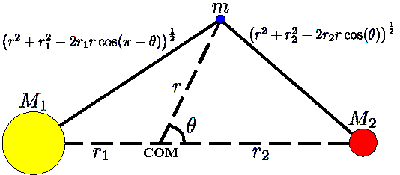Fig. 2: Variable definitions for inspection of L4/L5. Adapted from .

In this section, I will derive the locations of L4 and L5 in the restricted three-body problem, loosely following . It is convenient to switch to a reference frame rotating with frequency Ω about the center of mass of the two-body system consisting of the larger two masses. We take Ω as given by Kepler's Third Law for the two larger bodies: Ω2 = G(M1+M2)/R3, where M1 and M2 are the masses of the large bodies (possibly a star and a large planet), G is the gravitational constant, and R is the total distance between the two bodies. In the rotating system, we will use polar coordinates, (r,θ). See Fig. 2, and note that R = r1+r2.

In the rotating system, the effective force on a test mass of mass m at position r is then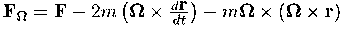where F is the gravitational contribution to the force and the other terms constitute Coriolis and centrifugal effects respectively. The generalized potential energy in the rotating frame is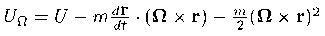Here U is the gravitational potential energy with F=-(grad)U.

The potential U as a function of the rotating polar coordinates can be determined from Fig. 2 using the law of cosines and Newton's Universal Law of Gravitation, yielding: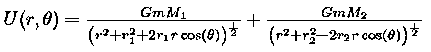For the equilibrium points in the rotating system, we note that the position r is fixed, so the Coriolis term is zero. Also, the effective force must be zero in both the r and &theta directions, yielding respectively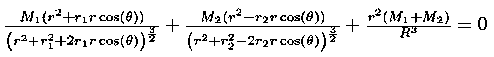and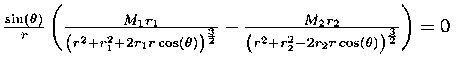We seek solutions off of the line joining the two larger masses (we seek L4 and L5, not L1, L2, or L3), thus sin(θ) &ne 0 and this term can be divided out of the second equation. Recalling from the definition of center of mass that M1r1 = M2r2, we can solve the second equation to find r cos(θ) = (r2-r1)/2. Thus, the L4 and L5 Lagrange points are equidistant from the two masses. Using this fact, the denominators of the first equation simplify (and are equal), giving us: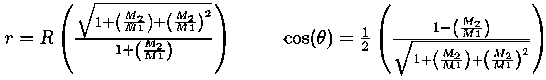In the limit M1 << M2, r approaches R and &theta approaches ±60 degrees. The test mass then orbits M1 at the same radius as M2, but leads or lags M2 by one-sixth of a period. For the case of Jupiter and the Sun, M2/M1 is about 0.00095. Then, the L4 and L5 points are at approximately 0.9995 times the orbital radius of Jupiter and ±58 degrees.

The stability of Lagrange Points L4 and L5 can be determined via linearization of the equations of motion [3,4]. They are stable if M2/M1 is less than approximately 0.04 . This is clearly the case for the Sun-Jupiter system, and would still hold for a much smaller star with a much bigger planet.

Possible Methods of Observing Extrasolar Trojan Planets

The above discussion should have made clear the possible existence of small extrasolar Trojan planets at the L4 or L5 points of the orbits of larger extrasolar planets. The existence of these Trojan planets would constrain some theories of the formation and development of planetary systems . Qualitatively, the methods for observing these extrasolar Trojans are quite simple. These methods draw heavily upon techniques which have already been used to find extrasolar planets in general.

There are three primary ways to observe an extrasolar planet, despite the fact that only the star about which it orbits can be seen directly. First, a sufficiently large planet may noticeably affect the motion of its host star. Since the relative radial velocity of the star with respect to an earth-observer can be measured accurately from its redshift, these perturbations of the star�s motion might be directly observable (see for instance ).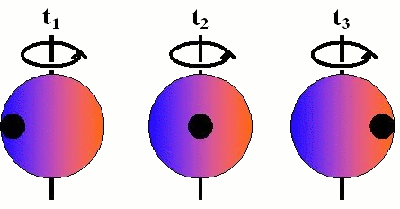Fig. 3: Illustration of the Rossiter-McLaughlin effect. The redshift of the photons emitted by the star varies with position as a result of the star's rotation. As the planet passes in front of the star (from time t1 to time t3), different spectroscopic features are observed because different regions of the star are blocked from view. Adapted from .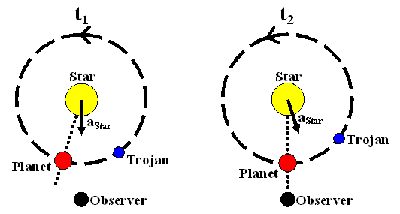Fig. 4: Illustration of the time lag between the minimum stellar radial velocity (at time t1) and the stellar eclipse by the primary planet (t2). The star's acceleration is not directly toward the planet due to the presence of a smaller Trojan. Since the star undergoes nearly uniform circular motion, its velocity is perpendicular to its acceleration. Thus, its speed along the observer's line of sight is minimized when the acceleration points towards or away from the observer, as at t1. Adapted from .

The remaining two methods for observing extrasolar planets result from eclipsing of the star by the planet. In terms of photometry, one would notice a dimming of the host star as the extrasolar planet passed in front of it . A second, more subtle, result of eclipsing is known as the Rossiter-McLaughlin effect . This effect can occur if the axis of rotation of the star is sufficiently skewed from our line of sight, as in Fig. 3. Then light from one side of the star will be slightly blueshifted away from the mean, while the light from the other side will be slightly redshifted. As the extrasolar planet passes from one side of the star to the other during an eclipse, it will thus preferentially block either the slightly redshifted or the slightly blueshifted light, temporarily altering the stellar spectral features observed from Earth.

All of these methods can be applied with slight modifications to the search for extrasolar Trojan planets. If the Trojan is sufficiently massive to affect the motion of its host star beyond the effects of the primary extrasolar planet, this might be observable as a direct effect on the star's radial velocity [5, 9]. Also, if the orbital plane of the Trojan planet is close to that of the primary extrasolar planet, then one might be able to observe one of the above eclipsing effects for both planets . In light of the above discussion of the locations of L4 and L5, one would expect the eclipse of the Trojan to precede or succeed that of the primary planet by one-sixth of a period.

Combinations of the extrasolar planet observation methods discussed above may be used even in more complicated situations with Trojan planets. For instance, a novel method has been suggested for the detection of a Trojan whose orbital plane does not coincide perfectly with that of the primary planet. In this case, the primary planet might eclipse the star, while the Trojan never does. The presence of the Trojan could then be inferred from a discrepancy between the times of eclipse of the planet and the minimum relative radial velocity of the star . This is shown in Fig. 4. It has also been shown that the presence of a terrestrial mass Trojan planet could be inferred from its small effects on the orbital period of the primary planet . These effects could be measured by one of the methods described above even with modern observational equipment. Thus, continued study of extrasolar planetary systems has the potential of revealing Trojan planets, thus constraining our theories of planetary system development.

© 2007 A.F. Sugarbaker. The author grants permission to copy, distribute and display this work in unaltered form, with attribution to the author, for noncommercial purposes only. All other rights, including commercial rights, are reserved to the author.

References

 H. Goldstein, C. Poole, and J. Safko, Classical Mechanics, 3rd Ed., (Addison-Wesley, 2002).

 N.J. Cornish, Lagrange Points (part of WMAP's education and outreach program), (2007/10/30)
(http://www.physics.montana.edu/faculty/cornish/lagrange.html).

 N.J. Cornish and J. Goodman, The Lagrange Points (part of WMAP's education and outreach program), (2007/10/30)
(http://www.physics.montana.edu/faculty/cornish/lagrange.pdf).

 J.V. Hosé and E.J. Saletan, Classical Dynamics: A Contemporary Approach, (Cambridge, 1998).

 E.B. Ford and M.J. Holman, Astrophys. J. Lett. 664, L51 (2007).

 E.B. Ford and B.S. Gaudi, Astrophys. J. Lett. 652, L137 (2006).

 J.F. Rowe, et al., Astrophys. J. 646, 1241 (2006).

 B.S. Gaudi and J.N. Winn, Astrophys. J. 655, 550 (2007).

 G. Laughlin and J.E. Chambers, Astron. J. 124, 592 (2002).